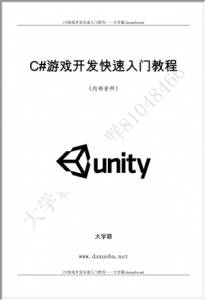# 减少代码的重复书写C#游戏开发快速入门大学霸

## 减少代码的重复书写C#游戏开发快速入门大学霸## 4.6  减少代码的重复书写减少代码的重复书写C#游戏开发快速入门大学霸

01     using UnityEngine;

02     using System.Collections;

03

04     public class MyScript : MonoBehaviour

05     {

06              private int number1;

07              private int number2;

08              private int number3;

09              private int number;

10              void Start()

11              {

12                       number1 = 3;

13                       number2 = 5;

14                       number3 = 4;

15                       number1 *= number1;

16                       number2 *= number2;

17                       number = number1 + number2;

18                       Debug.Log(“number1*number1 + number2*number2 = ” + number);

19

20                       number1 = 3;

21                       number2 = 5;

22                       number3 = 4;

23                       number1 *= number1;

24                       number3 *= number3;

25                       number = number1 + number3;

26                       Debug.Log(“number1*number1 + number3*number3 = ” + number);

27              }

28     }

01     using UnityEngine;

02     using System.Collections;

03

04     public class MyScript : MonoBehaviour

05     {

06              private int number1;

07              private int number2;

08              private int number3;

09              private int number;

10              void Start()

11              {

12                       number1 = 3;

13                       number2 = 5;

14                       number3 = 4;

15                       Debug.Log(“number1*number1 + number2*number2 = ” + AddTwoNumber(number1,number2));

16                       Debug.Log(“number1*number1 + number3*number3 = ” + AddTwoNumber(number1,number3));

17              }

19              {

20                       i1 *= i1;

21                       i2 *= i2;

22                       return i1 + i2;

23              }

24     }